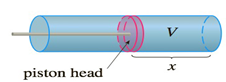Chapter 5.4, Problem 29E

Chapter
Section
Textbook Problem

# When gas expands in a cylinder with radius r, the pressure at any given time is a function of the volume: P = P ( V ) . The force exerted by the gas on the piston (see the figure) is the product of the pressure and the area: F = π r 2 P . Show that the work done by the gas when the volume expands from volume V 1 to volume V 2 is W = ∫ V 1 V 2 P   d VTo determine

To show:

The work done by the gas when the volume expands from volume V1 to volume V2 is

W=V1V2P dV

Explanation

1) Concept:

The work is calculated by using the formula W=abf(x)dx

2) Given:

The pressure is the function of volume P=P(V) and F=πr2P

3) Calculation:

Since the pressure P=P(V) is a function of volume, and the volume V=πr2x is a function of x, therefore, the pressure can be written as a function of x

Therefore, if V1=πr2x1 and V2=πr2x2 then the work done by the gas when the volume expands from volume V1 to volume V2 is

W=x1x2F(x) dx

Given F=πr2P

W=x1x2πr2P(V(x)) dx

Substitute (x)=πr2x, therefore,dV<

### Still sussing out bartleby?

Check out a sample textbook solution.

See a sample solution

#### The Solution to Your Study Problems

Bartleby provides explanations to thousands of textbook problems written by our experts, many with advanced degrees!

Get Started

#### In problems 63-73, factor each expression completely. 72.

Mathematical Applications for the Management, Life, and Social Sciences

#### The range of is: (−∞,∞) [0, ∞) (0, ∞) [1, ∞]

Study Guide for Stewart's Multivariable Calculus, 8th

#### For y = sin2 x + cos2 x, y = _____. a) 2 sin x 2 cos x b) 2 sin x cos x c) 4 sin x cos x d) 0

Study Guide for Stewart's Single Variable Calculus: Early Transcendentals, 8th